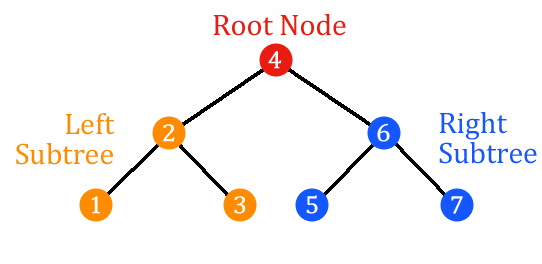# Inorder Traversal

Inorder traversal is a depth-first search algorithm for a binary search tree that first traverses the left subtree, then the root, then traverses the right subtree. This provides the nodes of the binary search tree in increasing order. To get nodes in decreasing order, inorder traversal can be done in reverse.

## Algorithm

The inorder algorithm can be described as follows:

``````Function Inorder(tree)
return Inorder(left-subtree) + root + Inorder(right-subtree)
``````

## Example

For the following binary search tree:Inorder traversal provides the nodes in the following order: `1`, `2`, `3`, `4`, `5`, `6`, `7`.